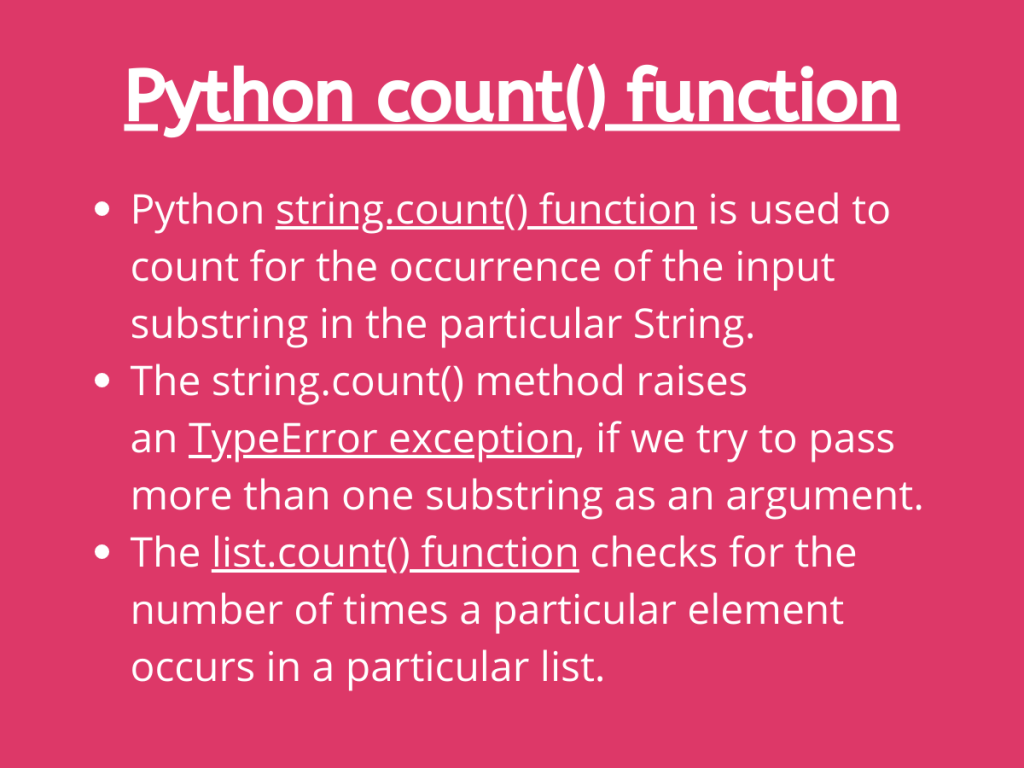# How to Use the Python count() FunctionHi, Folks! In this article, we will be focusing on Python count() method with Strings and Lists.

## 1. Python count() function with Strings

Python String has got an in-built function – string.count() method to count the occurrence of a character or a substring in the particular input string.

The `string.count() method` accepts a character or a substring as an argument and returns the number of times the input substring happens to appear in the string.

Syntax:

```string.count(string, start_index,end_index)
```
• `substring(mandatory)`: The string whose occurrence of presence needs to be counted in the input string.
• `start_index(optional)`: The index from where the search for the substring starts.
• `end_index(optional)`: The index where the search for the substring needs to stop.

Example:

```inp_str = "JournalDev -- AskPython @ JournalDev"
str_cnt = inp_str.count("JournalDev")
print(str_cnt)
```

Output:

```2
```

Example 2:

```inp_str = "Python Java Python Kotlin"
str_cnt = inp_str.count("Python", 0 , 6)
print(str_cnt)
```

In the above example, we have passed ‘Python‘ as a substring to be searched and to count for the presence between index 0 – index 6.

Output:

```1
```

Example 3:

```inp_str = "Python Java Python Kotlin"
str_len=len(inp_str)
str_cnt = inp_str.count("Python", 5 , str_len )
print(str_cnt)
```

Here, we search the substring – ‘Python’ and count its occurrence between index 5 to the end of the string that is why we have passed the length of the string as the end_index argument.

Output:

```1
```

### Python String count() method: TypeError

Python string.count() accepts only a single substring as an argument. If we try to pass multiple substrings as arguments, it raises the `TypeError exception`.

Example:

```inp_str = "Python Java Python Kotlin"
str_cnt = inp_str.count('Python', 'Java')
print(str_cnt)
```

Output:

```TypeError                                 Traceback (most recent call last)
<ipython-input-40-6084d1350592> in <module>
1 inp_str = "Python Java Python Kotlin"
----> 2 str_cnt = inp_str.count('Python', 'Java')
3 print(str_cnt)

TypeError: slice indices must be integers or None or have an __index__ method

```

## 2. Python List count() function

Python list has got a list.count() method to count the occurrence of particular elements in a list.

The `list.count() method` counts the occurrence of a particular value/ data item present in the input list.

Syntax:

```list.count(value)
```

Example 1:

```inp_lst = ['Apple','Banana','Apple','Grapes','Jackfruit','Apple']

lst_cnt = inp_lst.count('Apple')
print(lst_cnt)
```

Output:

```3
```

Example 2:

```inp_lst = [ ['Rat','Cat'], ['Heat','Beat'], ['Rat','Cat'] ]

lst_cnt = inp_lst.count(['Rat','Cat'])
print(lst_cnt)
```

In the above example, we basically count the occurrence of a nested list [‘Rat’, ‘Cat’] inside the list.

Output:

```2
```

## Python count() function at a glance!

• Python `string.count() function` is used to count for the occurrence of the input substring in the particular String.
• The string.count() method raises an `TypeError exception`, if we try to pass more than one substring as an argument.
• The `list.count() function` checks for the number of times a particular element occurs in a particular list.

## Conclusion

Thus, in this article, we have understood the working of in-built Python count function with Strings and Lists.

## References

• Python String count() function – JournalDev